# Understanding Principal Component Analysis and its Application in Data Science — Part 1

## Learn the mathematical intuition behind PCA

Principal Component Analysis (PCA), is a method used to reduce the dimensionality of large datasets. We will also study the covariance matrix and the multivariate normal distribution in detail since understanding them will result in a better understanding of PCA. The Python scripts in this article show you how PCA can be implemented from scratch and using the Scikit Learn library.

Notation

Currently Medium supports subscripts and superscripts only for some characters. So to write the name of the variables, I use this notation: Every character after ^ is a superscript character and every character after _ (and before ^ if it is present) is a subscript character. For example

is written as P_B^T in this notation. In this article, bold-face italic lower-case letters (like a) refer to vectors. Bold-face italic capital letters (like A) refer to matrices, and italic lower-case letters (like a) refer to scalars. Capital letters (like X) refer to random variables and bold-face capital letters (like X) refer to both random vectors. The numbers above an equality sign like refer to the number of the equations that were used to derive the expression on the righ-hand side of that equality sign.

Dimensionality reduction

Let’s start with a simple example. Suppose that we have a small dummy set with two features x and y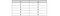Table 1

The dataset has two dimensions, and we can see that x and y depend on each other (x=y). This dataset is plotted in Figure 1, and the features x and y are represented by the axes x and y respectively.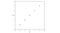Figure 1

Now suppose that we want to reduce its dimension to one so that we can describe it with only one feature. However, we want to do it with minimal loss of information. The first thing that we can try is simply removing one of the features. However, we lose lots of information about the spreading of data in this way. In this dataset, the distance between each two consecutive data points is the square root of 2. Suppose that we remove the second feature y and only keep x. Now the distance between each two consecutive data points along x is 1. So we are underestimating the spread of data points, and it happens since x and y depend on each other (I will explain the mathematical reason for that later).

We can try another method. Let’s define a new coordinate system as shown in Figure 2. Here one of the axes (u) passes through the data points and the other one (v) is perpendicular to that. In fact, the new coordinate system (u-v) is the result of the rotation of the original coordinate system (x-y) by 45 degrees.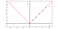Figure 2

Now we need to calculate the coordinates of the data points in the u-v coordinate system. To do that we project each data point onto each new axis. So, we have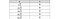Table 2

The features x and y are now replaced by features u and v. The value of v is not a function of u since it does not change by u. We say that u and v are independent of each other since if we change one of them, the other one won’t be affected. However, we still need to decide which one should be eliminated. Here it is clear that we should keep u. By eliminating v, we minimize the loss of information. Since u and v are independent, we are getting the correct distance between the consecutive data points, and the only piece of information that is lost is the v-coordinate of these points.

Now we can summarize the steps that we took to perform the dimensionality reduction. First, we need to define a new coordinate system (by rotating the original one) in which the transformed features are independent. Then we need to sort these features based on the amount of information that they hold and eliminate one or more features that are ranked lower than the others. PCA roughly follows the same procedure. The only difference is that it finds a coordinate system in which the features are uncorrelated. The difference between the uncorrelated and independent features will be explained later, but to understand them, first, we need to review some statistical concepts.

Random variables

A random variable is a variable whose numerical value depends on the outcome of a random phenomenon. So its value is initially unknown, but it becomes known once the outcome of the random phenomenon is realized. We commonly use uppercase letters to denote a random variable, and lowercase italic letters to denote the particular values that a random variable can assume.

For example, the two outcomes of tossing a coin are heads or tails. We can assign values to each outcome and denote getting heads by 0 and tails by 1. Now we can define the random variable X with possible values of X=0 or X=1. A particular value that X can take on is denoted by x. This is an example of a discrete random variable. A discrete random variable can only take certain values. On the other hand, a continuous random variable can take any values within an interval. For example, the outcome of measuring a person’s weight can be described by a continuous random variable. If X is a continuous random variable, the probability that X takes a value in the interval [a, b] can be written as

where f(x) is called the probability density function (PDF) of X. We know that

So the integral of a PDF over the entire space must be equal to 1

Mean

Let X be a continuous random variable that can take the value x with the PDF f(x). The mean or expectation of X, denoted by E(X), is a number defined as follows:

The most important property of mean is linearity. If X₁, X₂, …, X are n random variables and a₁, a₂, …, a and c are constants, then we can show that

The proof is given in the appendix.

Variance

Variance is a measure of the spread of the data around its mean. If X is a random variable then the variance of X, denoted by Var(X), is defined as follows:

If the random variable X takes a constant value, then its variance is zero, since X is always equal to its mean. On the other hand, the larger X deviates from its mean, the larger the variance of X is. The standard deviation of X, denoted by σX, is the nonnegative square root of Var(X) if the variance exists:

If a is a constant then we can show that

The prove it first we use Eq. 3 to get

Now we can use the definition of variance

Independence

Suppose that event Y has occurred and we wish to compute the probability of another event X taking into account that we know that Y has occurred. The probability of event X is denoted by P(X). The conditional probability of X is the probability of X given that Y has occurred and is denoted by P(X|Y). Two events are independent if the occurrence of one does not affect the probability of occurrence of the other. So if X and Y are independent then we can write

Eqs. 7 and 8 can be shown in another form using the Bayes’ rule. The Bayes’ rule states that

Where P(X, Y) is the joint probability of X and Y or the probability of X and Y occurring together. So using the Bayes’ rule we can say that two events X and Y are independent if and only if

If X and Y are two independent random variables, then it can be shown that

Covariance

Let X and Y be two random variables. The covariance of X and Y, which is denoted by Cov(X, Y) is defined as

The covariance of X and Y provides a measure of the association between X and Y. A positive covariance indicates that the deviations of X and Y from their respective means tend to have the same sign. So when one of them (X or Y) is high, the other one tends to be high, and when one of them is low the other one tends to be low. It also means that they tend to increase or decrease together or move in the same direction. On the other hand, a negative covariance indicates that the deviations of X and Y from their respective means tend to have opposite signs. When one of them is high, the other one tends to be low, and when one of them increases, the other one tends to decrease. So they tend to move in the opposite direction.

The covariance of a random variable with itself is equal to its variance:

We can also write the covariance in a different form. If X is a random variable and c is a constant then based on Eq. Equation 3 we have

Now we can use the fact that E[X] and E[Y] are constants and write

Now if X and Y are independent, we can use Eq. 11 and write

So two independent random variables have a zero covariance.

Correlation

The correlation between X and Y, denoted by ρ(X, Y), is defined as follows:

Based on Cauchy-Schwarz inequality we have

So we can write

And by dividing the previous inequality by σ_X σ_Y we get

Based on Eq. 16, the correlation and covariance have the same sign (since standard deviation is always positive). When ρ(X, Y)>0, X and Y are positively correlated and tend to move together, and when ρ(X, Y)<0, they are negatively correlated and tend to move in the opposite direction. If ρ(X, Y)=0, X and Y are said to be uncorrelated.

Besides, it can be shown that if there is a linear relationship between X and Y

where a and b are constants and a≠0, then we have

So we can write |ρ(X, Y)|=1. If a>0, then the line has a positive slope and X and Y should increase or decrease together. So we have a positive covariance and correlation and ρ(X, Y)=1. Similarly if a<0, X and Y move in the opposite direction, and ρ(X, Y)=-1. Finally, If we have two independent random variables, their covariance is zero (Eq. 15), so their correlation is zero and we say they are uncorrelated.

In summary, the value of ρ(X, Y) provides a measure of the extent to which two random variables X and Y are linearly related. If the values that X and Y take are relatively concentrated around a straight line with a positive slope, then ρ(X, Y) will be close to 1. On the other hand, if these values are relatively concentrated around a straight line with a negative slope, then ρ(X, Y) will be close to −1.

In Numpy we can use the function corrcoef() to calculate the correlation between two arrays. We can think of each array as a list of the observed values of a random variable. Suppose that we have two random variables X and Y. The observed values of X (denoted by x) are 19 evenly spaced numbers on the interval [0, 10]. So x={0, 1.5, 2, …, 10}. Besides, suppose that Y=X, so they have a linear relationship and y=x. Figure 3.a shows the plot of y vs x.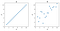Figure 3

We can now easily calculate the correlation between X and Y using Listing 1:

The output is:

The return value of corrcoef(x, y) is a 2-d array that can be thought of as a matrix defined as follows:

Using Eqs. Equation 5, Equation 13, and Equation 16 we can write

So the diagonal elements of this matrix are always equal to 1. From the definition of covariance, we can see that Cov(X, Y)= Cov(Y, X) and ρ(X, Y)= ρ(Y, X). Hence, the off-diagonal elements of this matrix are always equal and either of them gives the correlation between X and Y. So based on the output of Listing 1, the calculated correlation is equal to 1 which is the expected result. Now we add some noise to the y values:

Figure. Figure 3.b shows the plot of y vs x. We can calculate the correlation again:

Output

As you see the correlation is now ~0.8. It is less than 1 since all the data points don’t lie on a straight line anymore. However, they are relatively concentrated around a straight line with a positive slope, so ρ(X, Y) is close to 1.

Random vectors

Dimensionality reduction only applies to multi-dimensional data which can be represented by random vectors. A random vector is a vector of random variables. If we have n random variables X₁, X₂, …, X, we can place them in the random vector X:

We use bold uppercase letters to denote a random vector. To show a possible value of a random vector (which is also a vector) we use bold italic lowercase letters such as x. So a possible value of X is

In a random vector X, the probability that X₁ ∈ [a₁, b₁], X₂ ∈ [a₂, b₂], …, X_n ∈ [a_n, b_n] can be written as

where

is the joint probability density function of X. The integral of a joint PDF over the entire space must be equal to 1. If we have the joint PDF of the random vector X, we can derive the distribution of one of its components X using marginalization. The marginal probability density function of X can be derived from the joint PDF of X as follows

which means that we integrate the joint PDF with respect to all the variables except x. It can be shown that the random variables X₁, X₂, …, X are mutually independent if and only if the PDF of X can be written as

Let’s see an example. Suppose that X is a random vector with n elements. µ is a column vector with n elements, and Σ is an n×n matrix. We say that X has a multivariate normal distribution (MVN) if its joint PDF is defined as

where x is a possible value of X, and det(Σ) is the determinant of Σ. µ and Σ are the parameters of the MVN distribution (later we will discuss this distribution in more details). Now suppose that n=2, and we have a random a vector

with an MVN distribution. We can generate some possible values of X using NumPy’s function random.multivariate_normal(). We just need to determine the parameters of the distribution. Suppose that the parameters are:

In Listing 4, we generate and plot 150 random values of X. The result is plotted in Figure 4.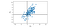Figure 4

Random vectors can describe the statistical nature of a dataset. A dataset is usually in the form of a table like Table 3. Each column of this table is called a feature of the dataset and we denote it by x, and each row is called a record that contains one observation for all the features. So, the dataset of Table 3 has n features and m records, and the value xᵢⱼ in this dataset is the observed value of feature j in the i-th record.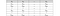Table 3

Now we can assume that the i-th feature is represented by the random variable X, and as usual, x denotes the possible values that X can take in general. In this dataset, the specific values of x are x_1i, x_2i ,… x_mi. If we place X₁, X₂, …, X in a random vector X, then X describes the whole dataset. Again, the possible values that X can take are denoted by

Each record of this dataset

is a specific value of x. If we could plot this dataset in an n-dimensional space, then each axis of this space represents one of the features and its corresponding random variable, so we can call the i-th axis, x, and each record of this dataset is an n-dimensional point in that space.

Random matrix

Similar to a random vector we can define a random matrix. A random matrix is a matrix whose entries are random variables. An m×n random matrix X can be written as

where each element Xᵢⱼ is a random variable.

Mean of a random vector

The mean of a random vector

is defined as:

Mean of random matrix

The mean of a random matrix X (Eq. 20) is defined as the matrix of the means of the elements of X:

If X is a random matrix, and A, B, and C are constant matrices then we have:

The proof is given in the appendix. Vectors can be considered as a matrix in which the number of columns is 1 (for row vectors the number of rows is 1). So Eqs. 22 and 23 are also valid for these vectors. If X is a random vector and a is a constant vector, then based on Eq. 22 we have

Where aᵀ is the transpose of a. The transpose of an m×n matrix A is an n×m matrix whose columns are formed from the corresponding rows of A. In fact, the (i, j) element of the transposed matrix is equal to the (j, i) element of the original matrix

So the transpose of a row vector becomes a column vector with the same elements and vice versa.

Variance of a random vector

Defining the variance of a multi-dimensional dataset is a little more difficult than defining its mean. The variance defined in Eq. 4 is based on a scalar random variable X. So, to use it for a random vector, we first need to convert this random vector to a scalar random variable, and we can use the scalar projection for this purpose.

Suppose that we want to calculate the variance of the random vector X along the vector u. The scalar projection of a random vector X onto another vector u is equal to

where||u|| is the length of u and is defined as

Since we only care about the direction of u we can assume that it is a unit vector, and its length is equal to 1:

So u.X=uᵀ X gives the scalar projection of X onto u. Now we can use Eq. 4 to calculate the variance of X along u which is equal to the variance of the scalar random variable uᵀ X:

Now if we have a dataset represented by X, each possible value that X can take is a vector x and is represented by a record in our dataset. If x has n elements, we can also think of x as a point in an n-dimensional space (Figure 5). In fact in an n-dimensional space, a point can be always represented by a vector. We first project all these vectors (or points) on u. So for each vector x, we have the scalar value uᵀ X and we can simply use Eq. 4 to calculate the variance of these scalar values (Figure 5).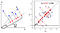Figure 5

The term in the square brackets can be simplified further. We know that

is a scalar (since the result of a dot product is a scalar quantity). Besides, we know that the transpose of a scalar quantity is equal to itself. So we can write

Finally, we know that for any vectors u and v

So we can write

Since u and uᵀ are constant vectors, we can use Eqs. 22 and 23 to have

where

is called the covariance matrix. So we can write the variance of X along u in terms of the covariance matrix:

Let’s take a more detailed look at the covariance matrix. If the random vector X has n element (Eq. 18), E[X] will have the same number of elements (Eq. 21). So X-E[X] is a column vector with n elements and (X-E[X]) is a row vector with n elements and by multiplying them, we get an n×n matrix (so S is a square matrix). If we do the multiplication we have:

Now using the definitions of variance and covariance we can write the previous equation as:

So the (i, j) element of the covariance matrix is the covariance of variables X and X and the i-th diagonal element gives the variance of X.

A symmetric matrix is a matrix that is equal to its transpose. So its (i, j) element is equal to its (j, i) element. We know that Cov(X, X)=Cov(X, X), so the covariance matrix is symmetric and is equal to its transpose:

Now let’s take a second look at the dataset plotted in Figure 4. We can calculate its mean using Eq. 21:

Output

We can also calculate its variance along the two arbitrary lines x₁=x₂ and x₁=-x₂. We first need the unit vectors along these lines which are

respectively. We can write

Output

Here we used np.dot() to calculate the dot product of the data points with u1 and u2 and np.var() to calculate the variance. The result shows that the spread of data along x₁=x₂ is much larger than the spread of data along and x₁=-x₂ (Figure 6).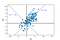Figure 6

Before we calculate the covariance of this random vector, we need to introduce the basis of a vector space.

Basis

A set of vectors {v₁, v₂, …, v} form a basis for the vector space V, if they are linearly independent and span V. When a set of vectors is linearly independent, it means that no vector in the set can be written as a linear combination of the other vectors. Mathematically, a set of vectors {v₁, v₂, …, v} is linearly independent if the vector equation

has only the trivial solution

A set of vectors span a vector space if every other vector in the space can be written as a linear combination of this set. So every value of the random vector X in V can be written as:

where a₁, a₂, …, a are some constants. The vector space V can have many different vector bases, but each basis always has the same number of vectors. The number of vectors in a basis of a vector space is called the dimension of that vector space. For now, you can assume that a vector with n-elements belongs to an n-dimensional vector space (with n basis vectors). But as we show later that is not always the case.

Orthonormal basis

Suppose that the random vector X belongs to an n-dimensional vector space V. A basis {v₁, v₂, …, v} is orthonormal when all the vectors are normalized (the length of a normalized vector is 1) and orthogonal (mutually perpendicular). When two vectors v and v are orthogonal their inner product is zero:

The length of v is

So for the orthonormal basis {v₁, v₂, …, v} we can write:

When the basis {v₁, v₂, …, v} is orthonormal, we can easily calculate the coefficients of Eq. 34. To get a, we can write

Now using Eq. 36, we have

So a is equal to the dot product of x and v or the scalar projection of x onto v. The main reason to find a basis for a vector space is to have a coordinate system for that. If the set of vectors B={v₁, v₂, …, v} form a basis for a vector space V, then every vector x in that space can be uniquely specified using those basis vectors:

And the coordinate of x relative to this basis B is:

The vectors:

form the standard basis for V (in e the i-th element is one and all the other elements are zero). These vectors form an orthonormal basis since we have

Covariance of random vectors

Suppose that we have the random vector

We know that the covariance matrix for X is given in Eq. 32. The covariance in Eq. 12 is defined based on two scalar random variables. So, if we have the random vector X, we need to convert it into two scalar random variables to be able to use Eq. 12 for it. We can use the scalar projection, but we need to project X onto two different vectors. The scalar projection of the whole dataset (which is represented by a random vector) onto each of these vectors results in a scalar random variable and using Eq.12 we can calculate the covariance of these two scalar random variables.

Suppose that we want to calculate the covariance of the random vector X using the unit vectors u and u (we only care about the direction of these vectors, so we assume they are both normalized). The scalar projection of X onto u and uⱼ will be u X and u X respectively. Now we can use Eq. 12 to calculate the covariance of them:

where we used the fact that the scalar quantity

is equal to its transpose. So based on Eq. 39, uᵀSu gives the covariance of the scalar projection of X onto uand the scalar projection of X onto u(Figure 7).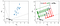Figure 7

We can easily show that:

To prove it, we use the fact that uᵀ Su is a scalar quantity, so we can write:

Now suppose that we have two scalar random variables X₁ and X₂. Their covariance is given by Eq. 12. Though these random variables are scalar, together they form a random vector

And the possible values of this random vector are

We can plot these values in a 2-d space. Then the horizontal and vertical axes represent x₁ and x₂ respectively. The vector X can be written in terms of the standard basis

where

and

So, X₁ and X₂ are the scalar projection of X onto e₁ and e₂ respectively, and we can write

As a result, if we combine the scalar random variables X₁ and X₂ as the random vector X, the covariance of them is the covariance of the scalar projection of X onto e₁ and e₂. This result is shown in Figure 8. Recall that the covariance in Eq. Equation 12 was initially defined for two scalar random variables. However, we can always combine them in a random vector and get the same result by calculating the covariance of that random vector along the vectors of the standard basis.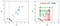Figure 8

The same result for an n-dimensional dataset can be obtained using Eq. 39. Let’s see what happens if we use two different vectors of the standard basis in Eq. 39. Using the defintion of covariance matrix (Eq. 32) we can write:

So eᵀSe gives the covariance of the random variables X and X or simply the (i, j) element of the covariance matrix. That is because each X is the scalar projection of X onto e. Now using Eqs. 39 and 40, the covariance of X and X can be written as

So each covariance element in the covariance matrix is the covariance of X along two different vectors taken from the standard basis. Besides, if i=j in Eq. 40, then we get

Change of basis

Suppose that we have a dataset with 2 features. This dataset can be represented with the random vector

where each X is a random variable that represents a feature of the dataset. As mentioned before, Each value of X

in this space can be written using the standard basis

So each x is represented by an axis of the coordinate system defined by the standard basis, and eᵀ Se gives the covariance of random variables X and X. However, we are not limited to the standard basis. Indeed, we can choose a different basis {u₁, u₂} with the constraint that these vectors are orthonormal. It is important to note that orthogonality is not a necessary condition for a basis, and we can have a basis in which the vectors are linearly independent but not orthogonal. However, in PCA, we only focus on the orthogonal bases.

By choosing the basis {u₁, u₂} we define a new coordinate system for X, and we can call the axes of this coordinate system x_B1 and x_B2. As Figure 9 shows this new coordinate is the result of the rotation of the original coordinate system. In the new coordinate system, u₁ and u₂ play the role of the standard basis and can be called e₁ and e₂. The random variables that represent the axes x_B1 and x_B2 are called X_B1 and X_B2 and can be placed in the random vector X_B.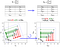Figure 9

By changing the coordinate system, the coordinate of each point in Figure 9 which can be generally written as x is changes to x_B. The features (x) change to (x_Bi). Their corresponding random variables changes from X to X_Bi, and the random vector that represents the whole dataset changes from X to X_B (Figure 9). The covariance matrix of the dataset also changes from S to S_B. If we have a basis B={u₁, u₂, …,u}, the matrix

is called the change-of-coordinate matrix. The columns of this matrix are the vectors in basis B. Now if we know the coordinate of the vector x in Rⁿ (which is simply x itself), the equation

gives its coordinate relative to basis B. We can also write

Let’s assume that in the new coordinate system, the axes are called x_Bi. The cooridate of a vector in the new coordinate system is written as

Now let’s focous on matrix P_B. A square matrix whose columns form an orthonormal set is called an orthogonal matrix, and the inverse of an orthogonal matrix is equal to its transpose. So if the basis B is orthonormal, then P_B is an orthogonal matrix and

It is important to note that the covariance of a random vector along two arbitrary vectors doesn’t change by changing the coordinate system. Suppose that we have a vector u in X, and after changing the coordinate system it is converted to u_iB in the new dataset represented by X_B. Now we can write

which means that the scalar projection of one vector onto another vector doesn’t change by changing the coordinate system (since it is just a distance). So using this equation we get

For example, in Figure 9, changing the coordinate system transforms u₁ and u₂ to e₁ and e₂ respectively. However, we have

A closer look at the covariance matrix

We can think of a matrix A as a transformation that acts on a vector x by multiplication to produce a new vector Ax. By looking at Eq. 39

we see that the covariance is the inner product of u and the vector Su. This vector is the result of the transformation of u by the covariance matrix. Let’s see what is the mathematical meaning of this transformation and what the vector Su represents? We start with vector space R² as an example. Assume that we have a circle that contains all the vectors that are one unit away from the origin. These vectors have the general form of

and the set of all such vectors is called D

Now we can calculate Su for all the vectors in this circle (the vectors in D). The set of all these vectors is called T.

These are the vectors in D which have been transformed by the covariance matrix S. The transformation matrix can change the direction and magnitude of the vectors in {u}. So generally, the original circle turns into an ellipse. Listing 7 shows an example of this transformation, and the result is shown in Figure 10.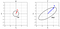Figure 10

A sample vector u₁ in D is transformed to t₁ and we have

The vector t₁ can have a different direction and magnitude than that of u (Figure 10). t₁ can be written in terms of the standard basis, however, we are free to choose a different basis. Here we choose an orthonormal basis in which one of the basis vectors is along u₁. The other one is perpendicular to it and we call it u₂. So, the new basis vectors are u₁ and u₂. Now we can write t₁ using these basis vectors

c₁ and c₂ are the scalar projections of t₁ onto u₁ and u₂, and to determine them, we can write

where we used the fact that the basis vectors form an orthonormal set, so they are normalized and perpendicular to each other. Now we can write

So we have

If we assume that S transforms u₂ into a vector named t

then we can similarly show that

So the vector Su₁ which is the result of the transformation of u₁ by the covariance matrix can be written as the sum of two vectors. One is along u₁ and its magnitude is equal to the variance of X along u₁, and the other is along u₂ (which is perpendicular to u₁), and its magnitude is equal to the covariance of X along u₁ and u₂ (Figure 11).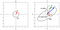Figure 11

This result can be extended to a dataset with n features which has an n×n covariance matrix. Let the set S be the set of all the other unit vectors in an n-dimensional vector space. Here we choose an orthonormal basis {u_1, u₂,…, u}. We also assume that

Now for each t we can write

And we have

So we can write

So by multiplying the covariance matrix with a unit vector u, we get a new vector. The components of this vector relative to an orthonormal basis that includes u, are the variance of X along u and the covariance of X along u and each of the other basis vectors.

Principal component analysis (PCA)

Now we are ready to discuss PCA. Let X be a random vector with n elements that represent our dataset. Each element of X is a random variable that describes a feature of the dataset. We want to find an orthonormal basis such that the correlation of the dataset along any pair of the vectors in this orthonormal basis is zero. The vectors of this basis are called the principal components of the dataset. If we think of this new basis as a new coordinate system for the dataset, then each basis vector defines a new axis for the dataset and represents a feature of the dataset in this coordinate system. So the goal of PCA is to find an orthonormal basis in which the features relative to this basis are uncorrelated (which means that the random variables that represent these features are uncorrelated).

Suppose that we have an orthonormal basis {u₁, u₂, …,u}. Remember that two random variables are uncorrelated when their covariance is zero. So the covariance of X along u and u for all different values of i and j should be zero

If we substitute Eq. 49 into Eq. 48, we get

for all the vectors u₁ to u. We know that the variance of X along u is a scalar. If we assume it is equal to λ, we can write the previous equation as

So when the covariance matrix transforms the vector u, it does not change its direction, and can only change its magnitude. This is not true for all the vectors in {u}. In fact, in each matrix like S, only some of the vectors have this property. These special vectors are called the eigenvectors of S and their corresponding scalar quantity λ is called an eigenvalue of A for that eigenvector. Generally, the eigenvector of an n×n matrix A is defined as a nonzero vector v such that:

where λ is an eigenvalue (eigenvalues and eigenvectors are only defined for square matrices). If we assume that the eigenvectors in Eq. 51 are normalized then each eigenvalue of the covariance matrix is equal to the variance of X along its corresponding eigenvector

The function numpy.linalg.eig() calculates the eigenvalues and eigenvectors of a square array in Numpy. For example, to calculate the eigenvalues and eigenvectors of the covariance matrix

We can write

Output

It returns a 1-d array (lam) which contains the eigenvalues and a 2-d array (v) that contains the normalized eigenvectors, such that the column v[:, i] is the eigenvector corresponding to the eigenvalue lam[i]. So for this matrix, we have

Listing 9 plots the transformed vectors of the covariance matrix and its eigenvectors. The result is shown in Figure 12.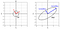Figure 12

It can be shown that an n×n real symmetric matrix has n linearly independent and orthogonal eigenvectors, and it also has n real eigenvalues corresponding to those eigenvectors (spectral theorem). So if we normalize the eigenvectors, they form an orthonormal set. It can be shown that in an n-dimensional vector space, a set of n linearly independent vectors form a basis for that space. So the set of the n eigenvectors of the n×n covariance matrix S (which is symmetric) is an orthonormal basis for an n-dimensional vector space. This is in agreement with our initial assumption that the basis for PCA should be orthonormal.

So far, we wrote Su₁ relative to an orthonormal basis in which one of the basis vectors is u₁ (others (u₂ to u) are perpendicular to u and each other). Now we can use the set of the eigenvectors as a new basis. Since the covariance matrix is n×n, the original vector u₁ belongs to an n-dimensional vector space, and we can use the set of the eigenvectors of the covariance matrix as a basis for that. Let’s call these eigenvectors with {v₁, v₂, …, v}. In fact {v₁, v₂, …, v} is a subset of D (the circle of unit vectors), and we only use different letters for them to distinguish these eigenvectors from the other vectors in D. Now we can write

where each c is the scalar projection of u₁ onto v (Figure 13).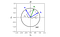Figure 13

If we assume that Su belongs to an n-dimensional vector space (as we see later this is not always the case) then we can write

If we substitute Eq. 53 into Eq. 54, we get

So Su can be written in terms of the eigenvectors (Figure 14 ).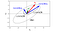Figure 14

Now the variance of X along u₁ can be written as (Eq. 31)

Since the set of eigenvectors are orthonormal, we can use Eq. Equation 36 to simplify the previous equation

We know that u₁ is a normalized vector, and its length is 1. Therefore, using Eq. 53 we can write

So, we have

As a result, in Eq. 57 the variance of X along u₁ is a weighted average of the variance of X along all the eigenvectors. As u₁ gets closer to one of the eigenvectors v, c increases (refer to Figure 13), and the weight for that eigenvector increases too. Suppose that we have sorted the eigenvectors based on their corresponding eigenvalues, so

is the greatest eigenvalue. Now in Eq. 57, the term on the right-hand side is maximized when λ₁ has the biggest weight in the average. So the variance of X along u₁ is maximized when c₁² = 1 and all other c are zero. This means that based on Eq. 53, u₁ is along the first eigenvector v₁. Hence the greatest variance of X is along v₁ and is equal to its corresponding eigenvalue

Now assume that we only focus on the vectors that are perpendicular to the greatest eigenvector (v₁). So for these vectors, there is no component for v₁, and in Eq. 53 we have c₁=0

Now in Eq. 57, λ₁ is absent, and the term on the right-hand side is maximized when λ₂ has the biggest weight in the average. This means that the variance of X along u₁ is maximized when c₂² = 1 and all other c are zero. Hence the maximum of the variance of X over all the vectors in D which are perpendicular to v₁ is along v₂ and is equal to its corresponding eigenvalue

Similarly, we can show that the maximum of the variance of X over all the vectors in D which are perpendicular to v₁, v₂, …, v₋₁ is along v and is equal to its corresponding eigenvalue

We can also calculate the length of the transformed vector t₁ using Eqs. 35 and 60

By following the same reasoning used for the Eq. Equation 57, we can show that the maximum of || Su₁ || over all vectors in D which are perpendicular to v₁, v₂, …, v₋₁ occurs at u₁= v and is equal to the corresponding eigenvalue of v

The ellipse shown in Figure 12 can be thought of as a circle stretched or shrunk along its principal axes. In fact, when we multiply the covariance matrix by the vectors of the unit circle, it transforms the unit circle by stretching or shrinking it along the principal components (Figure 15), and the principal axes of the ellipse (the major and minor axes) will be along these principal components.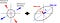Figure 15

The amount of stretching or shrinking along each principal component is proportional to its corresponding eigenvalue or the variance of the dataset along those principal components (Eq. 52). If the covariance matrix is the identity matrix

Then we have Su₁=u₁. So the covariance matrix doesn’t change the shape of the unit circle at all. If it is a scalar multiple of the identity matrix

Then we get Su₁=cu₁ for any vector u₁ in the unit circle. So, the result is a bigger or smaller circle but not an ellipse. Now using Eq. 46 we can also write

Where u₂ is perpendicular to u₁. We can get this result for any vectors u₁ in the unit circle. This means that if we choose two arbitrary orthogonal vectors, the covariance along them will be zero. Hence a covariance matrix which is a scalar multiple of the identity matrix represents a dataset in which the covariance along any pair of orthogonal vectors is zero. On the other hand, when the covariance matrix transforms the unit circle into an ellipse, it means that we have covariance along some vectors in the dataset.

Suppose that we have a covariance matrix in which all the covariance elements (non-diagonal elements) are zero, and the diagonal elements are different

This matrix still results in an ellipse. Recall that each covariance element in the covariance matrix is the covariance of X along two different vectors taken from the standard basis. Hence here the covariance of X along e₁ and e₂ is zero. Indeed e₁ and e₂ are the principal components, and the principal axes of the ellipse are along them. However, it is important to note that the covariance of X along any other pair of orthogonal vectors is not zero (Figure 16), and that is why we have an ellipse.

Finally, these results can be generalized to an n-dimensional space in which we have a unit n-dimensional sphere instead of the unit circle, and a covariance matrix which is not a scalar multiple of the identity matrix turns the unit n-dimensional sphere into an ellipsoid.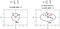Figure 16

Change of basis for the covariance matrix

Suppose that S is the covariance of the random vector X, and we want to choose a new basis B= {u₁, u₂, …, u_n} for X. What is the covariance of X relative to this new basis? First, we need to write X relative to the new basis. From Eqs. 43 and 44 we have

where

The same thing can be written for the mean of X

We can also write

Now using Eq. 30, the covariance matrix of X relative to basis B is

So we have

Now to get the (i, j) element of S_B, we can use Eq. 40

So, the covariance matrix relative to basis B is

If we have the original covariance matrix and the basis vectors, we can easily use this equation to calculate the covariance matrix relative to the new basis. Using Eq. 61, we can write

So we get

which means that we can decompose the covariance matrix relative to the standard basis to get the covariance matrix relative to a new basis.

Now let’s see what happens if we use the eigenvectors of the covariance matrix as a basis. Suppose that {v₁, v₂, …, v} are the eigenvectors of S, and their corresponding eigenvalues are {λ₁, λ₂, .., λ_n}. Let

Since these eigenvectors are the principal components, from Eqs. 49 and 52 we have

By substituting them into Eq. 62 we have

Eigendecomposition

As mentioned before, an n×n real symmetric matrix has n linearly independent and orthogonal eigenvectors, and we assumed that they are normalized, so these eigenvectors form an orthonormal set, and P_B is an orthogonal matrix. An n×n symmetric matrix A can be written as

where D is an n×n diagonal matrix comprised of the n eigenvalues of A. P is an orthogonal n×n matrix, and the columns of P are the n linearly independent and orthogonal eigenvectors of A that correspond to those eigenvalues in D respectively.

The decomposition of A based on Eq. 65 is called eigendecomposition. So in Eq. 64, the eigendecomposition of the covariance matrix gives the covariance matrix relative to the principal components as a new basis. If we simplify the right-hand side of Eq. 64 we get

Now if we multiply both of this equation by u₁ we have

Which gives Su₁ in terms of the eigenvectors and the terms in the brackets are components of Su₁ along each eigenvector. So the eigendecomposition of S gives the components of Su₁ along each eigenvector.

Design matrix

Now let’s see how we can implement PCA in Python. So far, we have used random vectors to derive the mathematical equations. But in practice what we have is a set of data points or observations, and we are going to see how we can apply the PCA analysis to these data points. Suppose that we have a dataset with n features and m datapoints

Each data point is a vector

which is a possible value for the random vector

that represents the dataset. We place all the datapoints in a matrix defined as

which represents the whole dataset and we call it the design matrix. In the textbooks, the design matrix is usually denoted by X. Here I use (it is read X tilde) to make it easier for the reader to distinguish it from a random vector. The i-th row of is the transpose of data point x, and the j-th column of gives the different values that the random variable X in X can take. Indeed the design matrix is the result of placing the elements of the data set in Table 3 into a matrix.

It is usual to center the dataset around zero before the PCA analysis. It means that we create a new dataset by subtracting the mean of X from X

which means that

Now we can see that the mean of X𝒸 is zero

This is like moving the origin to the mean of the dataset. The (i, j) element of the covariance matrix of X is

And the (i, j) element of the covariance matrix of X𝒸 is

which means that X and X𝒸 have the same covariance matrix, so their eigenvectors and eigenvalues will be the same. As a result, we can do the PCA analysis on X𝒸 instead of X and get the same results. From now we don’t use the subscript c to denote a centered dataset and simply use X for a centered dataset. Now suppose that our dataset {x₁, x₂, …, x} is already centered. Since the mean of this dataset is zero, we have

The (i, j) element of the covariance matrix for this dataset is

Each xᵢⱼ in this equation is the (i, j) element of . Here to get all the different values of X and X in the dataset, we get all the values of the i-th and j-th column of which are xₖᵢ and xₖⱼ for k=1..m. But to take the average we divide the sum by m-1, not m. That is because Cov(X, X) is the sample covariance which is used as an estimate of the population covariance. By dividing by m-1 we, make sure that we get an unbiased estimate of the population covariance. Now we can write the previous equation as

By using the definition of matrix multiplication we get

Please note that this equation is only valid when the dataset used to generate is centered. Let’s use PCA for a real dataset. We use the same dataset that we created in Listing 4. We should first center it and calculate (which is called Xtil in Listing 10). This listing shows the original dataset versus the centered dataset (Figure 17).

We can now calculate the covariance matrix using

Output

We can also use the function cov() in Numpy for this purpose. However, it accepts the transpose of Xtil as its argument

We also need to calculate the eigenvalues and eigenvectors of the covariance matrix.

Output

Each column of v is an eigenvector. These eigenvectors form a new orthogonal basis. To get the coordinate of the data points relative to this basis we project them onto the basis vectors in Listing 13. Only the values for the first 10 data points are shown here.

Output

Listing 14 plots the original dataset, the transformed vectors of the unit circle by the covariance matrix, and the projection of data points onto the principal components. The result is shown in Figure 18.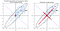Figure 18

We can also use the Scikit-learn library to do PCA. We can use the original dataset in Listing 4 (before centering) since it automatically centers the dataset before doing the PCA analysis. First, we need to give it the data points

The argument n_components gives the number of principal components that we want to use during the calculations. We usually don’t need all of them since we want to project our data points onto a lower dimension space. For example, if our dataset is 4 dimensional, it will have 4 principal components, but we can only keep the first two ones that have the highest eigenvalues. If n_components is not set all components are kept. We can use the explained_variance_ property to see the eigenvalues

Output

The output is a 1-d array that gives the sorted eigenvalues. So the first element is the greatest eigenvalue. Remember that each eigenvalue of the covariance matrix is equal to the variance of X along its corresponding eigenvector (Eq. 52). That is why this property is called the explained variance. We can also use the components_ property to get the principal components (eigenvectors).

Output

The output is a 2-d array. Each row of this array gives a principal component of the dataset. The i-th row (pca.components_[i, :]) gives the corresponding eigenvector (principal component) of the i-th element of pca.explained_variance_. You may have noticed that the eigenvectors reported by this property are in the opposite direction of the eigenvectors that were obtained in Listing 12. For any matrix A, If v is a corresponding eigenvector of λ, then any multiplier of v like cv is also the corresponding eigenvector of λ.

So in this case, if we call the eigenvectors obtained in Listing 12, v₁ and v₂ then pca.components_ returns -v₁ and -v₂ as eigenvectors. Finally, to get the scalar projection of the data points onto the principal components, we can use the transform() method.

Output

It returns a 2-d array, and the data points in this array have the same index of the data points in the design matrix passed to pca.fit(). So the i-th row corresponds to the i-th data point in the i-th row of points array. The j-th column gives the scalar projection of the data points onto the j-th principal component (remember that the principal components are sorted based on their eigenvalues). In fact, each row of this array gives the coordinates of a data point relative to the basis defined by the principal components. Here we only showed the first 10 rows of this array.

If you compare the results of Listing 16 with the ones obtained in Listing 13, you will notice that their absolute value is the same but they have opposite signs. That is because our basis is now -v₁ and -v₂. Indeed, the outputs of Listing 13 and 16 are still referring to the same projected points (red dots in Figure 18). We can reconstruct the vector x that represents the i-th data point using these coordinates. Since the principal components are a basis for the dataset, we can write

where {v₁, v₂, …, v} are the principal components, and c is the scalar projection of the xᵢ onto the j-th principal component. For example, the first data point in the design matrix in Listing 10 is represented by the vector

Output

The principal components are returned by pca.components_ and the coordinates of this vector are given by pca.transform(points)[0,:]. So to reconstruct this vector we can write

Output

which returns the same vector.

The rank of the covariance matrix (optional)

Suppose that we have a date set with n features, so the number of columns of the design matrix is n and the covariance matrix will be n×n (Eq. 68). As mentioned before an n×n symmetric matrix has n real eigenvalues, and n linearly independent and orthogonal corresponding eigenvectors (spectral theorem). So far we assumed that these n eigenvectors are the principal components of the dataset, and form an orthonormal basis for that. Indeed we assumed that a dataset with n features belongs to an n-dimensional vector space. As we will see in this section this is not always true.

Let’s go back to the first dataset that we saw at the beginning of this article (Table 1). In that dataset, all the data points are along the line y=x. We can now easily determine its principal components and eigenvalues.

We can get the covariance matrix for this dataset

Output

The eigenvalues are

Output

and the eigenvectors or principal components are

Output

So the first eigenvalue is 5 and its corresponding eigenvector is [-0.708 -0.708]. This is a unit vector along the line x=y. The second eigenvalue is a tiny number, and we can assume it is zero (In fact it should be zero, but we get a tiny number since we are estimating it with a numerical method). Figure 19 shows how the vectors in the unit circle are transformed by the covariance matrix of this dataset.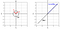Figure 19

As you see T={Su} is not an ellipse any more. It is now a straight line. Since the second eigenvalue is zero, it means that the data points have no variance along the second eigenvector. So the second principal axis is of no importance and we can ignore it. Here the first eigenvector v₁ = [-0.707, -0.707] spans all the vectors in T, the basis of T has only one vector which is v₁. The dimension of T is 1 since the columns of S are not linear independent. In this example, If we call the columns of S, c₁, and c₂ respectively, then we have c₁=c₂. So we get

Generally, an m×n matrix A does not necessarily transform an n-dimensional vector u into an m-dimensional vector Au. The dimension of the transformed vector Au can be lower if the columns of that matrix are not linearly independent. The column space of matrix A written as Col A is defined as the set of all linear combinations of the columns of A. If the columns of the matrix A are c, c, … c, then for any vector like u, Au can be also written as

So Au is a linear combination of the columns of A, and Col A is the set of vectors that can be written as Au. The number of basis vectors of Col A or the dimension of Col A is called the rank of A. Indeed, the rank of A is the dimension of {Au}.

The rank of A is also the maximum number of linearly independent columns of A. That is because we can write all the dependent columns as a linear combination of these linearly independent columns. Since Au is a linear combination of all the columns, then it can be written as a linear combination of these linearly independent columns. Hence, these linearly independent columns span Au and form a basis for col A, and the number of these vectors becomes the rank of A. In the previous example, the rank of S is 1.

But why in this example all the columns of the covariance were not linearly independent? If we print the centered design matrix, we get

Output

Here you see that the columns of the design matrix are equal, and they are not linearly independent. It can be shown that for a real matrix A, the rank of A and Aᵀ A are equal (refer to the appendix for proof), so according to Eq. Equation 68, the rank of S andshould be equal.

The rank of is also equal to the dimension of the random vector X that it represents. Here is the proof. Let X be a random vector with n elements, and x be a possible value that it can take. Now the n-dimensional standard basis can span x.

Now suppose that one of the elements of x is dependent on the other one. For example x₂ = cx₁. This means that in our all data points the second feature is equal to the first feature multiplied by c, and in the design matrix, the second column is equal to c times the first column. Now we can write the previous

Here we used a linear combination of e₁ and e₂ (e₁+ce₂) as a new basis vector. Since e₁ and e₂ were linearly independent of the other basis vectors, a linear combination of them will be also linearly independent of them. So now we have (n-1) linearly independent vectors that span x. Hence these (n-1) vectors form a basis for x and the dimension of x will be n-1.

As a result, when one column of the design matrix () becomes dependent on the others, the dimension of X is decreased by one, and the number of the remaining linearly independent columns is equal to the dimension of X.

If you have a design matrix in which the number of rows (m) is smaller than the number of columns (n), then the rank of the matrix will be m at most. A dataset with m elements is represented by the random vector X with m elements. This random vector can be spanned with the vectors of the standard basis with m elements

So we have m linearly independent vectors here. If all of them are needed to span your vector space, the dimension of X will be m. If you can combine some of them (like what we did in Equation 69 ), the dimension will be smaller than m. So the dimension of X and the rank of the design matrix cannot be greater than m.

If the number of rows (m) is greater than the number of columns (n) then the rank of the design matrix will be n at most since this is the maximum number of linearly independent columns that the matrix can have.

There is an important relationship between the rank of a square matrix and the number of its zero eigenvalues. For a symmetric matrix, it can be shown that the rank of the matrix equals the number of its nonzero eigenvalues (proof is given in the appendix). Since the covariance matrix is symmetric, we can use this theorem for that. In our previous example, we had two eigenvalues and one of them was non-zero, so the rank of S was 1.

So let me summarize the discussion. The covariance matrix is a symmetric matrix that has n linearly independent eigenvectors. The number of non-zero eigenvalues of the covariance matrix, gives us the rank of the covariance matrix, the rank of the design matrix, and the dimension of the random vector that represents our dataset.

The dimension of this random vector is not necessarily equal to the number of its elements or the number of features that we have in the dataset. The number of data points in the dataset can also limit the dimension of this random vector.

As an example, suppose that we have a few data points with 3 features, but we only include 2 of them in the design matrix. Based on the previous discussion the rank of the covariance matrix cannot be greater than 2 here.

Output:

But you see two eigenvalues are zero and the dimension of the dataset is only one (since we have only one non-zero eigenvalue). But why we get this result? Here we only have two data points and two points can be connected with a straight line. If we center the dataset, this line passes through the origin which means that the vector along this line is a basis vector for the dataset (Figure 20).

This is the eigenvector that has a non-zero eigenvalue and both the data points can be spanned by it (the blue vector in Figure 20). This example clearly shows the importance of the data points. The only thing that PCA sees is the data points that we sample from the random vector. If these data points are not a representative sample of that vector, PCA will underestimate its dimension.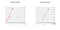Figure 20

I hope that you enjoyed reading this article. In the 2nd part of this article, a case study of the application of PCA in dimensionality reduction will be presented. In addition, the application of singular value decomposition (SVD) in PCA, the relation between the covariance matrix and multivariate normal distribution, and the limitations of PCA will be discussed. Please let me know if you have any suggestions.

You can find the second part of this article here: Understanding Principal Component Analysis and its Application in Data Science — Part 2

All the Code Listings in this article are available for download as a Jupyter notebook from GitHub at: https://github.com/reza-bagheri/PCA_article

Appendix

Properties of mean

1-If X₁, X₂, …, X_n are random variables and a_1, a_2, …, a_n and c are constants, then we can show that

Proof: We can first show that this is true for n=2:

where we have used marginalization in (a), and we used the fact that the PDF f(x₁, x₂) integrates to 1. Using this result, you can easily show that Eq. A.1 is true for each positive integer n by an induction argument.

2-If X is an m×n random matrix, and A is a constant m×n matrix:

Proof:

3-If X is an m×n random matrix, B is a constant k×m matrix and C is a constant n×p matrix:

Proof: For Eq. A.3 we can write:

The proof for Eq. A.4 is similar. If we combine the results of part 1 and 2, we can write

Similarly, we have

The rank of a symmetric matrix

We want to show that the rank of a symmetric matrix equals its number of nonzero eigenvalues. The null space of a matrix A is the set of all vectors x such that Ax=0. The nullity of A is the dimension of the null space of A. The rank theorem states that for an m×n matrix A

where n is the number of columns of A. Based on the spectral theorem, an n×n symmetric matrix B has n real eigenvalues, and n linearly independent corresponding eigenvectors. However, these eigenvalues are not necessarily distinct and some of the eigenvectors can have the same eigenvalues. Here we count the eigenvalues separately even if they are equal. So when we say the number of zero eigenvalues, it means the number of eigenvectors with a zero corresponding eigenvalue.

Now the nullity of B is the set of vectors v such that Bv=0 which can be also written as Bv=0v. So the eigenvectors of B with a zero eigenvalue form the null space of B, and since these eigenvectors are linearly independent, they can form a basis for the null space of B, so the number of eigenvectors with a zero eigenvalue or simply the number of zero eigenvalues is the nullity of B. Now based on the rank theorem we can write

rank B=n-nullity A=n-number of zero eigenvalues=number of nonzero eigenvalues

and since the total number of eigenvalues is n we get

The rank of A and Aᵀ A are equal

Let A be a real m×n matrix, and x be a real vector with n elements. First, we will show that

If Ax=0, we can multiply both sides by Aᵀ to get Aᵀ Ax=0

Now assume that

and let y=Ax, so we get Aᵀ y=0. By multiplying both sides of the previous equation by Xᵀ we have

Using the definition of y and Eq. 28 we get

Based on its definition, y has m elements

So using Eq. A.4 we can write

Since A and y are real matrices, y will be real too, and yᵀ y is a sum of positive real numbers. So the previous equation implies that

And y=Ax=0. So we just proved that all the vectors x that make Ax=0 make (Aᵀ A)x=0 and vice versa. So A and Aᵀ A have the same null space and hence the same nullity. We know that both Aᵀ A and A have n columns. Now based on The rank theorem (Eq. A.5) we have

Since the nullity of Aᵀ A and A are equal, their ranks will be equal too.

## More from Reza Bagheri

Data Scientist and Researcher. LinkedIn: https://www.linkedin.com/in/reza-bagheri-71882a76/